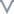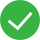# 了解IF ELSE 解决场景中的一些问题

``````IF 条件 THEN 返回值1 ELSE 返回值2

``````

``````if [地区] in("东北","华北" ) then  "北方"  else [地区]
``````

``````if [年龄]>=0 and  [年龄]<10 THEN "0-10岁"   else if [年龄]>=10 and  [年龄]<20 THEN "10-20岁"  else if  依次类推
``````

``````if [地区]="东北" then [销售额]
``````

``````if [地区]="001" then "东北" else if [地区]="002" then "华北"  else [地区]
``````

``if isnull([地区]) or  [地区]=" " then "Y" ELSE "N"``根本没帮助文档较差文档一般文档不错文档很好

#### 更多建议

0/200